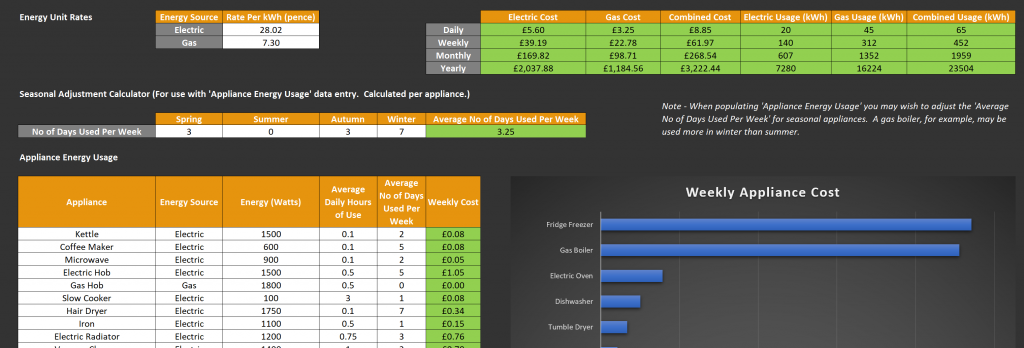This blog contains a free downloadable energy cost calculator for gas and electricity in the UK, including custom rate input, daily/weekly/monthly/yearly cost forecasts and real-time charting.

## Gas and Electricity in the UK

Energy is sold to you by a price per kilowatt hour (kWh).  This is also known as a unit rate.  And appliances that we use in our home are rated for power in watts (W) or kilowatts (kW).

To calculate the costs of running these appliances we can multiply the power output of each appliance, by the unit rate and then by the amount of time we are running the appliance for.  This will give us an indication of how much energy we are using, and the cost.

Obtaining costs and energy usage per appliance is an approximation – we can approximate that we’ll use our oven for half an hour per day, but that isn’t guaranteed of course!  Hence in our calculator we try and specify an average time per day, and an average number of days per week that we use each appliance.We can also use the seasonal adjustment calculator to factor in different seasons.   For example, in the winter we might have the central heating on every day.  Whereas in summer we might never have it on!  We can use the season adjustment calculator to work out an average number of days we use an appliance per week, throughout the four seasons.

## Electricity Calculator and Gas Calculator

You can download the Microsoft Excel electricity calculator and gas calculator below.  We have populated it with sample values.  You may wish to search the internet for the specific model of your appliances to find the correct power output,  and then fine tune the usage times.  If you notice any discrepancies or ways to improve the calculator, please comment below!

## Energy Cost Calculator

Energy Cost Calculator for Gas and Electricity in the UK.